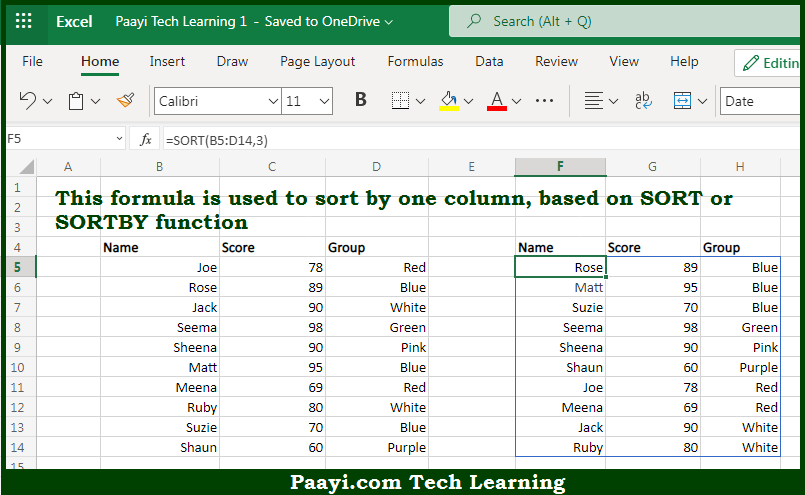# Learn How to Sort by One Column in Microsoft Excel

Written by | 0 Comments | 547 Views

In this article, you will learn how to evaluate things in Dynamic Arrays with formulas in Microsoft Excel using a single/combination(s) of functions. You will also know How to Sort by One Column and see the generic formula.

Learn How to Sort by One Column in Microsoft Excel

The main purpose of this formula is to sort by one column. Here we will learn how to sort by one column in the given data range in the workbook in Microsoft Excel. That implies, with the help of a formula based on the SORTBY and SORT functions you can able to sort by one column. So, with the help of this formula, you can able to sort by one column in the given data range in the workbook in Microsoft Excel.

General Formula to Sort by One Column

=SORT(data,index,order)

The Explanation to Sort by One ColumnSo we know that with the help of the given formula above you can able to sort by one column. Here we will learn how to sort by one column in the given data range in the workbook in Microsoft Excel. As we know that the SORTBY function allows the sorting based on one or more "sort by" arrays, as long as dimensions are compatible with the source data. In the example given here, we are unable to use the named range "custom" directly in SORTBY. So, with the help of this formula, you can able to sort by one column. So now you have learned how to sort by one column, in the given data range in the workbook in Microsoft Excel.